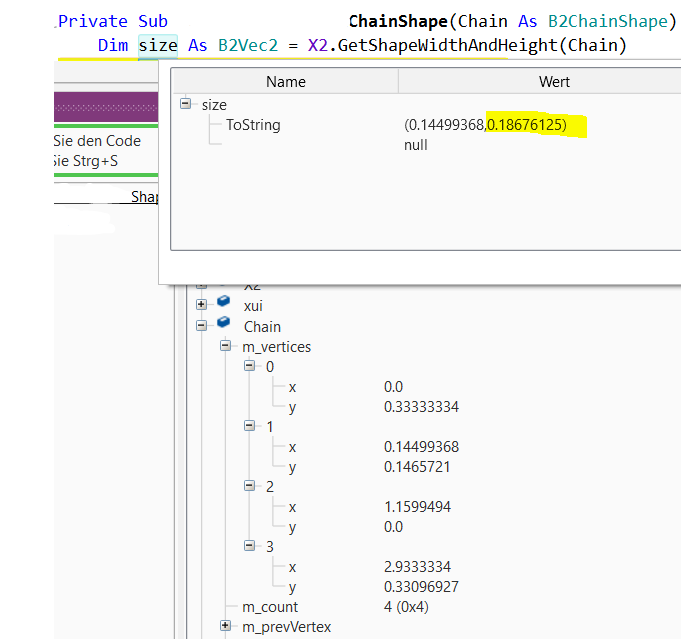# GamesStrange Values returned by GetShapeWidthAndHeight<->ChainShape

#### Gunther

##### Active Member
Longtime User
Please have a look to the sizing values of a ChainType Shape.

It seems that the Shape Width and Height are not correct returned by the function.

The X value is the one from the first Edge vertice and the Y value is a moon value.

According to the actual values in that chain is should be something like ( 5.96 , 0.66 ).

The chain values are read via Tiled into the app.

Possible bug or not the best approach of mine?#### Erel

##### B4X founder
Staff member
Longtime User
GetShapeAndWidth shouldn't be used with chain shapes. Only with polygon, rectangle or circle shapes.

•Gunther

#### Gunther

##### Active Member
Longtime User
Ok, how I get savely the dimentions then for a chain shape?

#### Erel

##### B4X founder
Staff member
Longtime User
Untested code:
B4X:
``````Dim edge As B2EdgeShape
edge.Initialize(Null, Null)
chain.GetEdge(0, edge)
Dim BottomLeft, TopRight As B2Vec2
BottomLeft.X = Min(edge.Vertex1.X, edge.Vertex2.X)
TopRight.X = Max(edge.Vertex1.X, edge.Vertex2.X)
BottomLeft.Y = Min(edge.Vertex1.Y, edge.Vertex2.Y)
TopRight.Y = Max(edge.Vertex1.Y, edge.Vertex2.Y)
Dim aabb As B2AABB
aabb.Initialize2(BottomLeft, TopRight)
For i = 1 To chain.EdgeCount - 1
chain.GetEdge(i, edge)
aabb.BottomLeft.X = Min(aabb.BottomLeft.X, Min(edge.Vertex1.X, edge.Vertex2.X))
aabb.TopRight.X = Max(aabb.TopRight.X, Max(edge.Vertex1.X, edge.Vertex2.X))
aabb.BottomLeft.Y = Min(aabb.BottomLeft.Y, Min(edge.Vertex1.Y, edge.Vertex2.Y))
aabb.TopRight.Y = Max(aabb.TopRight.Y, Max(edge.Vertex1.Y, edge.Vertex2.Y))
Next``````
aabb.Width and Height store the width and height.